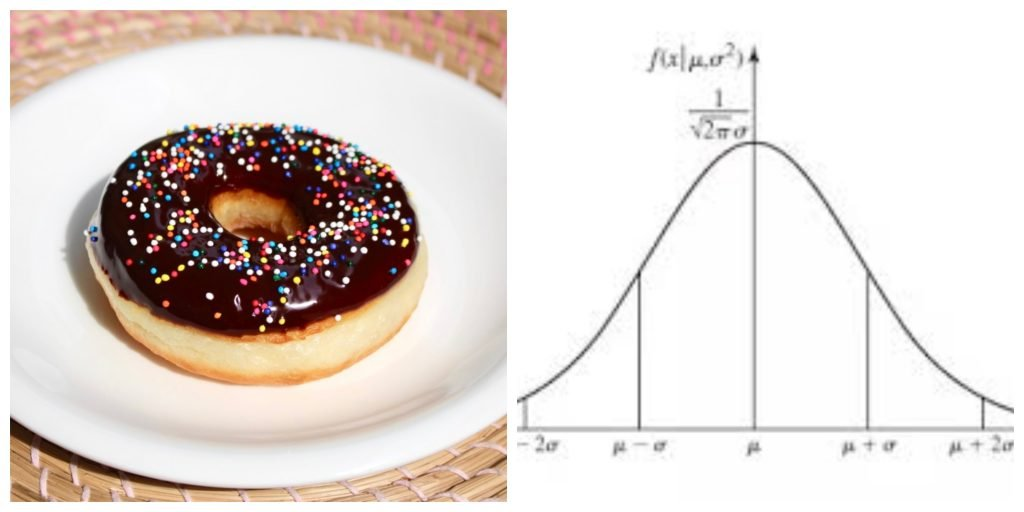Get inspired by the success stories of our students in IIT JAM MS, ISI  MStat, CMI MSc Data Science.  Learn More

# ISI MStat 2016 Problem 10 | PSB Sample | It's a piece of cake!This is a sample problem from ISI MStat 2016 Problem 10, which tests the student's ability to write a model and then test the equality of parameters in it using appropriate statistics.

## ISI MStat 2016 Problem 10:

A cake weighing one kilogram is cut into two pieces, and each piece is weighed separately. Denote the measured weights of the two pieces by $X$ and $Y$ . Assume that the errors in obtaining $X$ and $Y$ are independent and normally distributed with mean zero and the same (unknown) variance. Devise a test for the hypothesis that the true weights of the two pieces are equal.

## Prerequisites:

1.Testing of Hypothesis

2.Model formation

## Solution:

Let us write the two cases in the form of a model:

$X= \mu_1 + \epsilon_1$

$Y = \mu_2 + \epsilon_2$

where, $\mu_1,\mu_2$ are the true weights of the two slices and $\epsilon_1 , \epsilon_2 \sim N(0, \sigma^2)$ (independently).

So, you get $X \sim N(\mu_1,\sigma^2)$ and $Y \sim N(\mu_2, \sigma^2 )$.

Also, see that $X,Y$ are independent.

So, we need to test $H_0: \mu_1=\mu_2 =\frac{1}{2}$ against $H_1: \mu_1 \neq \mu_2$.

See that, under $H_0$, $X-Y \sim N(0,2 \sigma^2)$

So, $\frac{X-Y}{\sqrt{2} \sigma} \sim N(0,1)$.

But have you noticed that $\sigma$ is unknown? So this isn't a statistic after all.

Can you replace $\sigma$ by an appropriate quantity so that you can conduct the test?

## Food For Thought:

Okay, let's move from cakes to doughnuts!!

Yeah, I know this is off topic and nothing related to statistics but it's good for the brain to alter cuisines once a while!

This is the famous doughnut slicing problem:

What is the largest number of pieces you can slice a doughnut into using only 3 cuts? (Note that you can only make planar cuts and you are not allowed to rearrange the pieces between the cuts)

I would request you to try this on your own without looking up solutions directly.

This is a sample problem from ISI MStat 2016 Problem 10, which tests the student's ability to write a model and then test the equality of parameters in it using appropriate statistics.

## ISI MStat 2016 Problem 10:

A cake weighing one kilogram is cut into two pieces, and each piece is weighed separately. Denote the measured weights of the two pieces by $X$ and $Y$ . Assume that the errors in obtaining $X$ and $Y$ are independent and normally distributed with mean zero and the same (unknown) variance. Devise a test for the hypothesis that the true weights of the two pieces are equal.

## Prerequisites:

1.Testing of Hypothesis

2.Model formation

## Solution:

Let us write the two cases in the form of a model:

$X= \mu_1 + \epsilon_1$

$Y = \mu_2 + \epsilon_2$

where, $\mu_1,\mu_2$ are the true weights of the two slices and $\epsilon_1 , \epsilon_2 \sim N(0, \sigma^2)$ (independently).

So, you get $X \sim N(\mu_1,\sigma^2)$ and $Y \sim N(\mu_2, \sigma^2 )$.

Also, see that $X,Y$ are independent.

So, we need to test $H_0: \mu_1=\mu_2 =\frac{1}{2}$ against $H_1: \mu_1 \neq \mu_2$.

See that, under $H_0$, $X-Y \sim N(0,2 \sigma^2)$

So, $\frac{X-Y}{\sqrt{2} \sigma} \sim N(0,1)$.

But have you noticed that $\sigma$ is unknown? So this isn't a statistic after all.

Can you replace $\sigma$ by an appropriate quantity so that you can conduct the test?

## Food For Thought:

Okay, let's move from cakes to doughnuts!!

Yeah, I know this is off topic and nothing related to statistics but it's good for the brain to alter cuisines once a while!

This is the famous doughnut slicing problem:

What is the largest number of pieces you can slice a doughnut into using only 3 cuts? (Note that you can only make planar cuts and you are not allowed to rearrange the pieces between the cuts)

I would request you to try this on your own without looking up solutions directly.

This site uses Akismet to reduce spam. Learn how your comment data is processed.

### One comment on “ISI MStat 2016 Problem 10 | PSB Sample | It's a piece of cake!”

1.Partha pratim das says:

Replace sigma by s

### Knowledge Partner